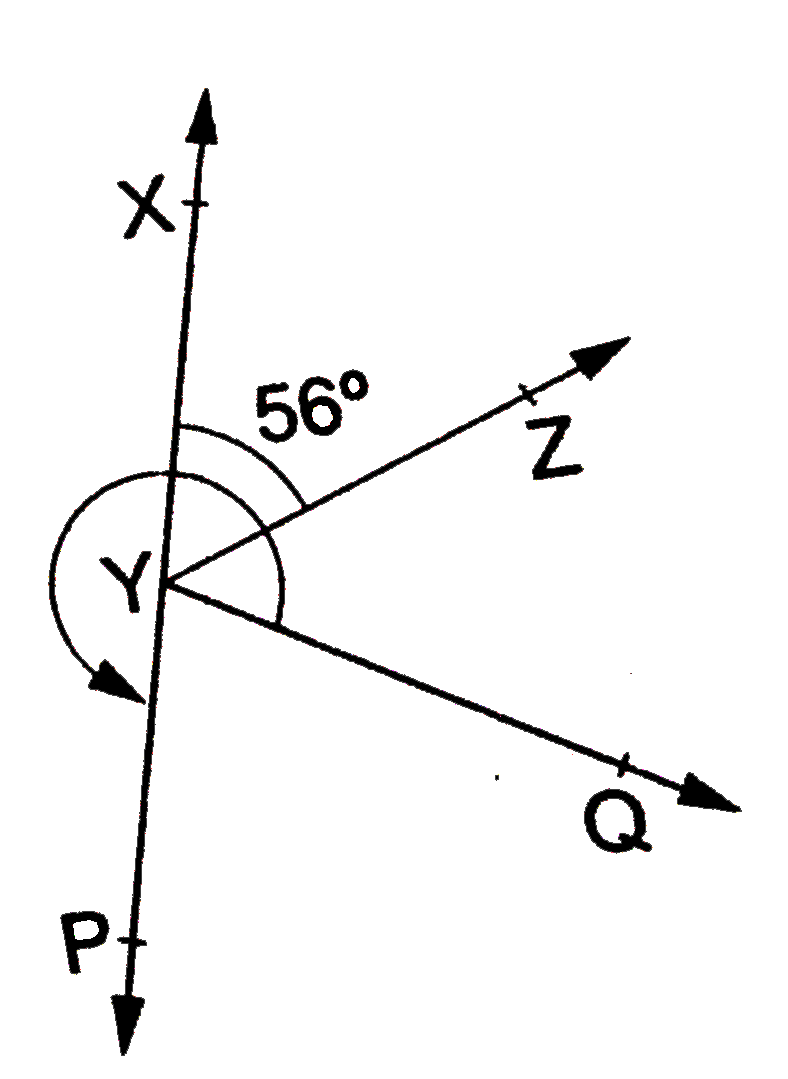# In the given figure, angleXYZ=56^(@) and XY is produced to a point P. If ray YQ bisects angleZYP, find angleXYQ and reflex angleQYP.

379 views

closed
In the given figure, angleXYZ=56^(@) and XY is produced to a point P. If ray YQ bisects angleZYP, find angleXYQ and reflex angleQYP.by (88.7k points)
selected by

Since XY is produced to point P, it follows that XP is a straight line and ray YZ stands on it.
:. angleXYZ+angleZYP=180^(@)
implies 56^(@)+angleZYP=180^(@)
implies angleZYP=(180^(@)-56^(@))=124^(@)
implies angleZTQ=1/2 angleZTP=(1/2xx124^(@))=62^(@).
:. angleXYQ=angleXYZ+angleZYQ=(56^(@)+62^(@))=118^(@).
Also, angleQYP=1/2 angleZYP=(1/2xx124^(@))=62^(@)
:. reflex angleQYP=360^(@)-angleQYP=(360-62)^(@)=298^(@).